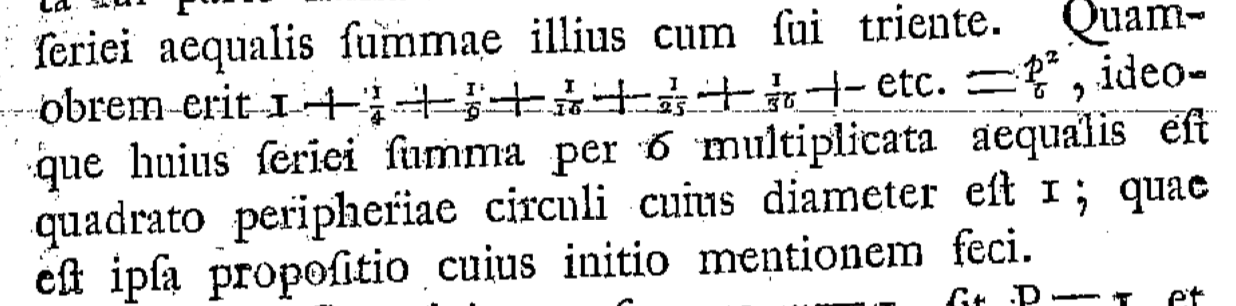# Why did Euler consider the zeta function?

Many zeta functions and L-functions which are generalizations of the Riemann zeta function play very important roles in modern mathematics (Kummer criterion, class number formula, Weil conjecture, BSD conjecture, Langlands program, Riemann hypothesis,...).

Euler was perhaps the first person to consider the zeta function $\zeta(s)$ ($1\leq s$). Why did Euler study such a function? What was his aim?

Further, though we know their importance well, should we consider that the Riemann zeta function and its generalizations happen to play key roles in modern number theory?

• I think his primary goal was to solve the so-called Basel problem. Having managed to do so, he probably wanted to study the values of the zeta function further. – Sylvain JULIEN Jun 29 '18 at 7:09
• Thank you very much. Basel's casual question leads to the modern number theory!! – Jojo Jun 29 '18 at 7:33
• @Jojo Basel is a city located in Switzerland, not a person... It's Euler's hometown. – Najib Idrissi Jun 29 '18 at 7:43
• Sorry. It is Mengoli who consider the Basel problem lol – Jojo Jun 29 '18 at 7:59

This history is described in Euler and the Zeta Function by Raymond Ayoub (1974). In his early twenties, around 1730, Euler considered the celebrated problem to calculate the sum $$\zeta(2)=\sum_{n=1}^\infty \frac{1}{n^2}.$$ This problem goes back to 1650, it was posed by Pietro Mengoli and John Wallis computed the sum to three decimal places. Ayoub conjectures that it was Daniel Bernoulli who drew the attention of Euler to this challenging problem. (Both lived in St. Petersburg around 1730.)

Euler first publishes several methods to compute the sum to high accuracy, arriving at $$\zeta(2)=1.64493406684822643,$$ and finally obtained $\pi^2/6$ in 1734. (We know this date from correspondence with Bernoulli.) It was published in 1735 in De summis serierum reciprocarum.$1+\frac{1}{4}+\frac{1}{9}+\frac{1}{16}+\frac{1}{25}+\frac{1}{36}+\text{etc.}=\frac{p^2}{6}$, thus the sum of this series multiplied by 6 equals the square of the circumference of a circle that has diameter 1. [Notice that the symbol $\pi$ was not yet in use.]

The generalization to $\zeta(s)$ with integers $s$ larger than two followed in "De seribus quibusdam considerationes". In 1748, finally, Euler derives a functional equation relating the values at $s$ and $1-s$ and conjectures that it holds for any real $s$. (Euler's functional equation is equivalent to the one proven a century later by Riemann.).

• @Jojo there was no reason to expect the central role the generalizations of $\zeta(s)$ would play in modern number theory back in the 1700s. The first time there was any generalization, to Dirichlet $L$-functions, was in the 1830s (and then only for real $s > 1$). Zeta-functions of number fields were introduced by Dedekind in the 1870s (but were only analytically continued to $\mathbf C$ by Hecke in 1917). The central role for these constructions and more like them (for Artin representations, modular forms, etc.) was largely a 20th century realization. – KConrad Jun 29 '18 at 15:02
• What did Euler think it meant, to relate $\zeta$ at $s$ and $1-s$, given that at least one of these doesn't converge? – Dan Piponi Jun 29 '18 at 15:35
• @DanPiponi --- for that reason Euler compared $s$ and $1-s$ for the alternating zeta function $\sum_n (-1)^{n}/n^s$ – Carlo Beenakker Jun 29 '18 at 15:49
• We should think it is key because of Euler’s product formula (also discussed in the Ayoub paper). – Francois Ziegler Jun 29 '18 at 16:09
• @DanPiponi, objecting to the lack of convergence at $s$ or $1-s$ is anachronistic. For someone like Euler in the 1700s, series were manipulated freely even if they make no sense to us. However, as Carlo says, Euler did use the alternating zeta-function (with terms $(-1)^{n-1}/n^s$, not $(-1)^n/n^s$, so it starts with $1$ at $n = 1$), which makes sense for $0 < s < 1$ but is strictly nonsense when ${\rm Re}(s) \leq 0$. My 2nd answer (not the accepted one) at mathoverflow.net/questions/13130/… shows how Euler found the functional equation. – KConrad Jun 29 '18 at 21:33